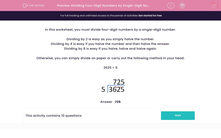# Dividing Four-Digit Numbers by Single-Digit Numbers (1)

In this worksheet, students divide a four-digit number by a single-digit number.This content is premium and exclusive to EdPlace subscribers.Key stage:  KS 2

Curriculum topic:   Maths and Numerical Reasoning

Curriculum subtopic:   Mixed Problems

Difficulty level:#### Worksheet Overview

In this worksheet, you must divide four-digit numbers by a single-digit number.

Dividing by 2 is easy as you simply halve the number.

Dividing by 4 is easy if you halve the number and then halve the answer.

Dividing by 8 is easy if you halve, halve and halve again.

Otherwise, you can simply divide on paper or carry out the following method in your head.

3625 ÷ 5